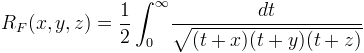Computes the Carslon elliptic integral RF.

Namespace:  Meta.Numerics.Functions
Assembly:  Meta.Numerics (in Meta.Numerics.dll) Version: 4.1.4Syntax
```public static double CarlsonF(
double x,
double y,
double z
)```

#### Parameters

x
Type: SystemDouble
The first parameter, which must be non-negative.
y
Type: SystemDouble
The second parameter, which must be non-negative.
z
Type: SystemDouble
The third parameter, which must be non-negative.

#### Return Value

Type: Double
The value of RF(x,y,z).Exceptions
ExceptionCondition
ArgumentOutOfRangeExceptionx, y, or z is negative.Remarks

The Carlson F integral is:As can be seen from the definition, RF is symmetric with respect to permutations among its parameters.

The Carlson integrals can be used to express integrals of rational functions. In that sense, they are replacements for the Legendre elliptic functions.See Also